# Basic Concepts in Information Theory Cheng Xiang Zhai

• Slides: 13Basic Concepts in Information Theory Cheng. Xiang Zhai Department of Computer Science University of Illinois, Urbana-Champaign 1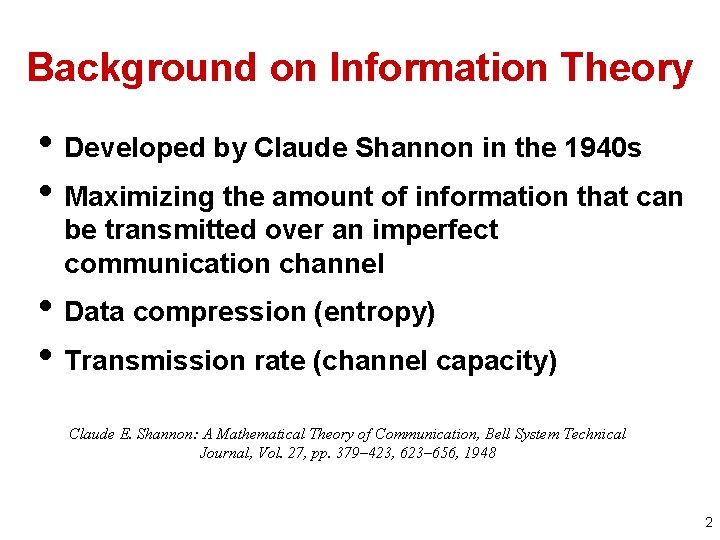Background on Information Theory • Developed by Claude Shannon in the 1940 s • Maximizing the amount of information that can be transmitted over an imperfect communication channel • Data compression (entropy) • Transmission rate (channel capacity) Claude E. Shannon: A Mathematical Theory of Communication, Bell System Technical Journal, Vol. 27, pp. 379– 423, 623– 656, 1948 2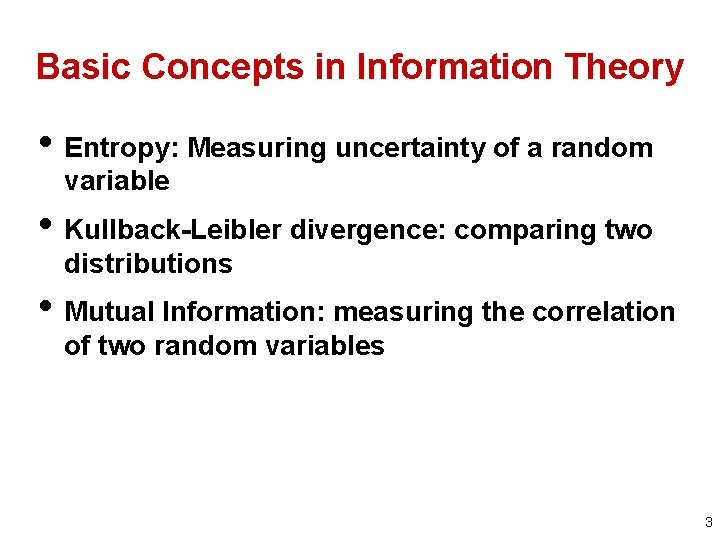Basic Concepts in Information Theory • Entropy: Measuring uncertainty of a random variable • Kullback-Leibler divergence: comparing two distributions • Mutual Information: measuring the correlation of two random variables 3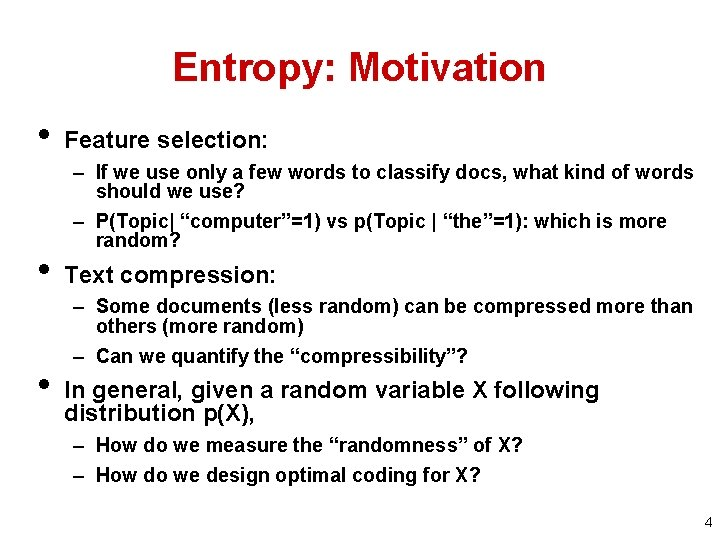Entropy: Motivation • • • Feature selection: – If we use only a few words to classify docs, what kind of words should we use? – P(Topic| “computer”=1) vs p(Topic | “the”=1): which is more random? Text compression: – Some documents (less random) can be compressed more than others (more random) – Can we quantify the “compressibility”? In general, given a random variable X following distribution p(X), – How do we measure the “randomness” of X? – How do we design optimal coding for X? 4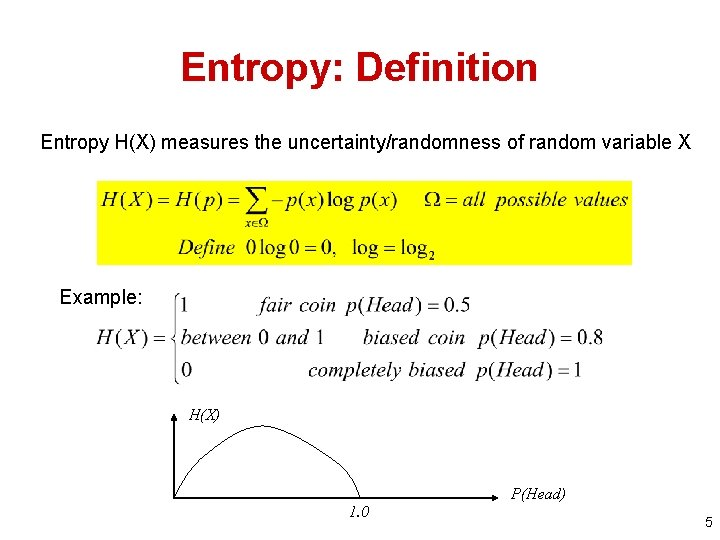Entropy: Definition Entropy H(X) measures the uncertainty/randomness of random variable X Example: H(X) P(Head) 1. 0 5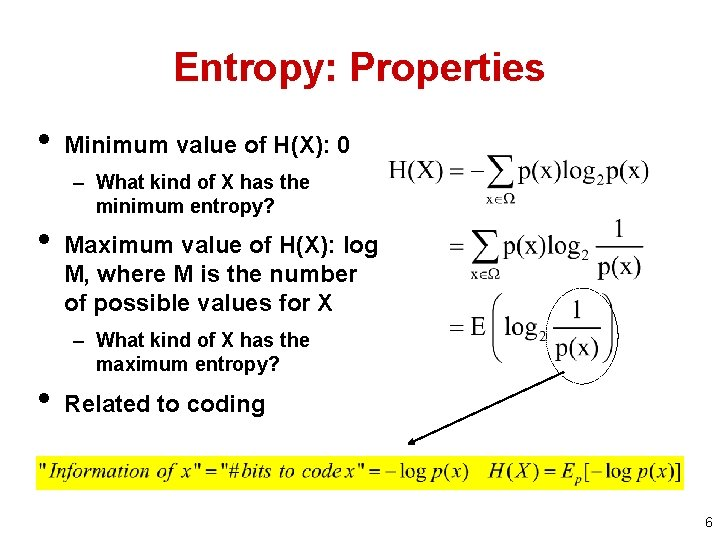Entropy: Properties • Minimum value of H(X): 0 – What kind of X has the minimum entropy? • Maximum value of H(X): log M, where M is the number of possible values for X – What kind of X has the maximum entropy? • Related to coding 6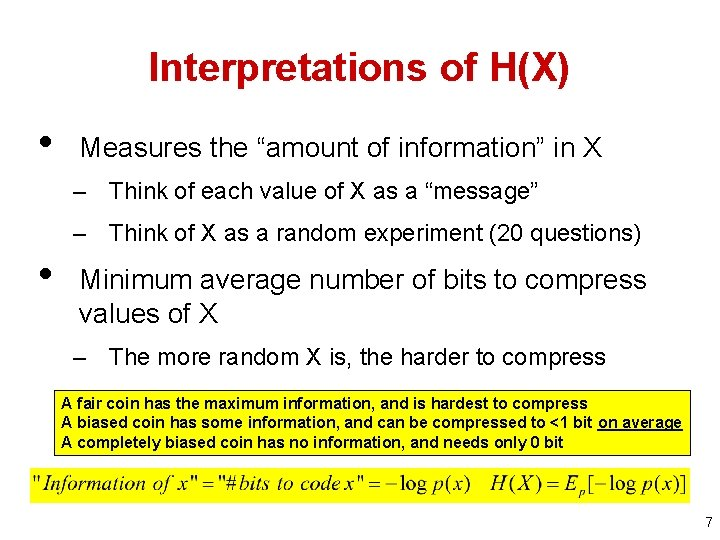Interpretations of H(X) • Measures the “amount of information” in X – Think of each value of X as a “message” – Think of X as a random experiment (20 questions) • Minimum average number of bits to compress values of X – The more random X is, the harder to compress A fair coin has the maximum information, and is hardest to compress A biased coin has some information, and can be compressed to <1 bit on average A completely biased coin has no information, and needs only 0 bit 7Conditional Entropy • • The conditional entropy of a random variable Y given another X, expresses how much extra information one still needs to supply on average to communicate Y given that the other party knows X H(Topic| “computer”) vs. H(Topic | “the”)? 8Cross Entropy H(p, q) What if we encode X with a code optimized for a wrong distribution q? Expected # of bits=? Intuitively, H(p, q) H(p), and mathematically, 9Kullback-Leibler Divergence D(p||q) What if we encode X with a code optimized for a wrong distribution q? How many bits would we waste? Properties: - D(p||q) 0 - D(p||q) D(q||p) - D(p||q)=0 iff p=q Relative entropy KL-divergence is often used to measure the distance between two distributions Interpretation: -Fix p, D(p||q) and H(p, q) vary in the same way -If p is an empirical distribution, minimize D(p||q) or H(p, q) is equivalent to maximizing likelihood 10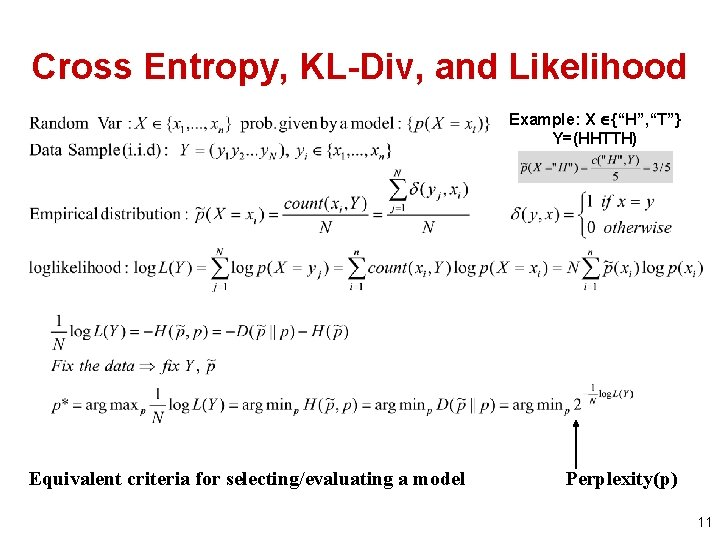Cross Entropy, KL-Div, and Likelihood Example: X {“H”, “T”} Y=(HHTTH) Equivalent criteria for selecting/evaluating a model Perplexity(p) 11Mutual Information I(X; Y) Comparing two distributions: p(x, y) vs p(x)p(y) Properties: I(X; Y) 0; I(X; Y)=I(Y; X); I(X; Y)=0 iff X & Y are independent Interpretations: - Measures how much reduction in uncertainty of X given info. about Y - Measures correlation between X and Y - Related to the “channel capacity” in information theory Examples: I(Topic; “computer”) vs. I(Topic; “the”)? I(“computer”, “program”) vs I(“computer”, “baseball”)? 12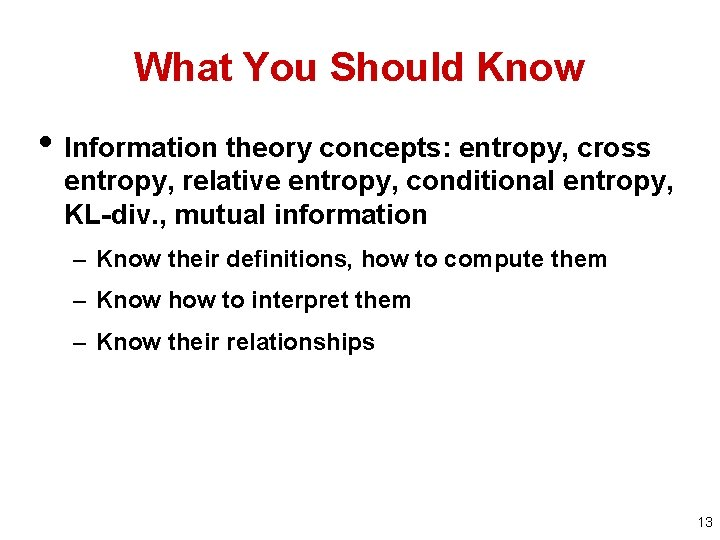What You Should Know • Information theory concepts: entropy, cross entropy, relative entropy, conditional entropy, KL-div. , mutual information – Know their definitions, how to compute them – Know how to interpret them – Know their relationships 13